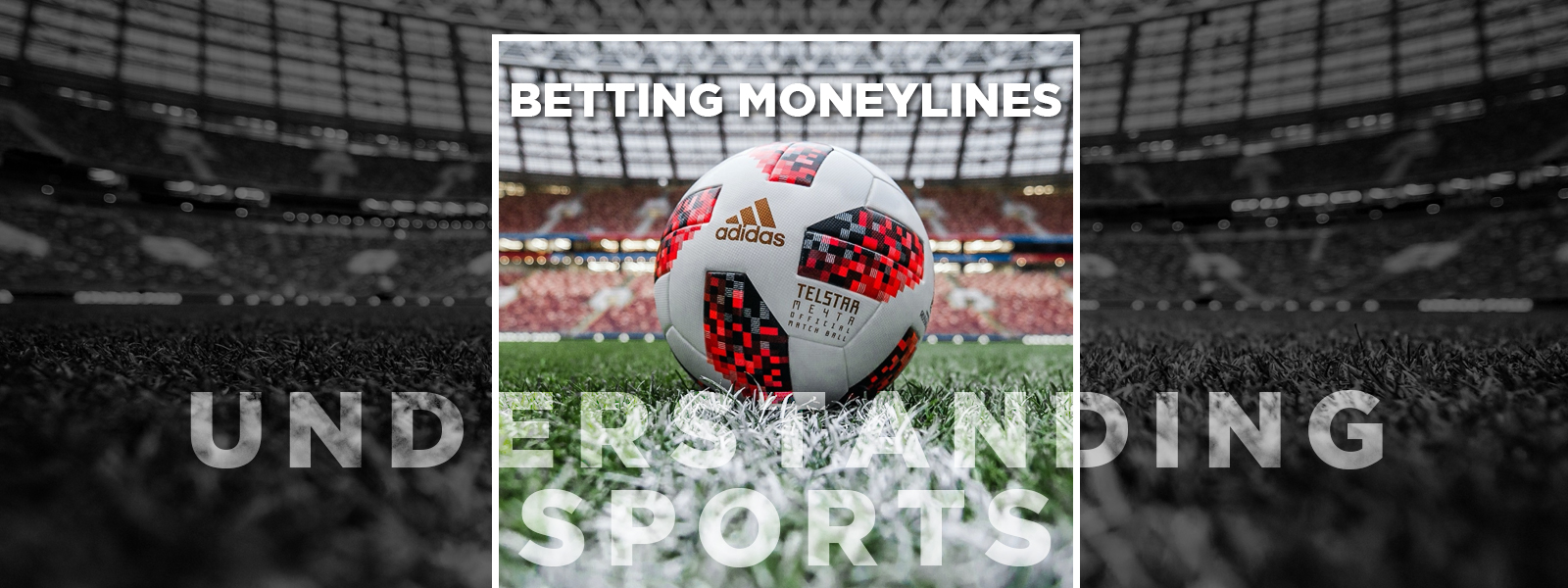Blog
Understanding Sports Betting Moneyline
Written by, Marko Vrakela Mon 15 Mar
Understanding Sports Betting Moneyline## Sports Betting Moneyline

Moneyline is the term used for a prevalent type of bet in North America. If you place a Moneyline bet, you are betting that one team will win a game. Unlike betting on a point's margin or spread, the winning margin does not affect your winnings. This type of wager is the equivalent of 1X2 bets used in Europe for the match-winner betting market. Odds for Moneyline bets are generally shown through a negative number and a positive one. The favorite team takes a negative number. The value of this number is the amount you would have to bet to get a \$ 100 net profit. For example, if the odd is -120 and you bet \$ 100, you will get \$ 220 back if the team you chose wins. The positive number represents the non-favorite or underdog. It shows the amount you would win if you bet \$ 100. If the line is +180, and you bet \$ 100, you will receive \$ 280 if your team wins. This does not mean that we should always bet \$ 100. You can invest the money you want, and your profit will be proportional to that amount.

Several factors contribute to the determination of the line for each team. The recent performance of each team or player is an essential factor when calculating the lines. Other elements such as player injuries or line-up changes can also affect odds. Odds seek to reflect each team's winning probabilities. Therefore, when betting on Moneyline, it is easy to know which is the favorite side and the underdog. The higher odds assigned to underdogs reward bettors for taking a higher risk. On the contrary, the favorite will present a lower odd since, in theory, he has more than 50% of winning probabilities. Another important aspect of handling Moneyline bets is knowing how to calculate the odds' implicit probability. To do this, it is first necessary to convert the odds given in American format to decimal format. You can do this conversion by merely dividing the profit between the stake and adding 1 to the result. That is, you must use the following formula:

• Odds = (Net profit / Stake) + 1

Once the odd has been transformed to decimal format, it will be enough to invert its value (divide one by the odd) and multiply it by 100. In this way, we will finally obtain the implicit probability value. Let's see a calculation example:

• New Orleans Saints -120 (favorite) will have a \$ 100 profit with a \$ 120 stake.
• The decimal odds would be: (100/120) + 1 = 1.83
• And the implied probability: 1 / 1.83 x 100 = 55%
• San Francisco Giants +145 (underdog) will have a \$ 145 profit with a \$ 100 stake.
• The decimal odds would be: (145/100) + 1 = 2.45
• And the implied probability: 1/2.45 x 100 = 41%

For many bettors, the direct way Moneyline odds refer to stake and profit is intuitive. So, this format eases understanding the market. The good thing is that we can know how much money we'll win or lose without having to do any math calculation.

recent post
categories
tags
Betting Articles